Home    About Us    Contact Us PUZZLES DIGITAL PUZZLES ACTIVITY MARKETS BrainSnack® Kakuro How to solve Kakuro? To guarantee the quality of our BrainSnack® Kakuro puzzles we made our own program in .NET C#, that only use these 6 human solvable logical strategies and mathematics, to generate the puzzles. You can use these strategies to solve them. 1. Solitary box If you only have to fill in one more box then deduct the filled in boxes from the sum. For example: 11-2-5 = 4.2. Unique common candidate Stand on the intersection of two runs and compare the combinations of the across run and the down run. All numbers that appear in both runs are candidates for that box. The combination for the across run 16 in 2 for box A is 79 and that of the down run 11 in 3 is 128, 137, 146, 236, 245 or the next series of unique numbers 12345678. If we compare the numbers of both runs then we see that there is only one common candidate, namely 7. Box A = 7.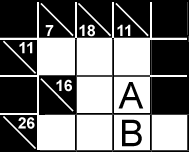3. Reducing runs The combinations for the across run 26 in 4 for box B are 2789, 3689, 4589, 4679 and 5678 or the following candidates: 23456789. In the down run (11 in 3) for B, we have already found the number 7 in box A. So we can reduce this run to a sum of 4 (11-7) in two boxes. For 4 in 2 there is only one combination 13. If we compare this with the across run for B we discover 3 as a unique common candidate. Box B= 3. 4. Deduction The across run for A, B and C is 20 in 3: this results in the following candidates 3456789. The down run for A and C is 16 in 2. The common candidates for A and C are therefore 79. The possible solutions for this run are 749 or 947. In other words B = 4.5. Limited area When you can demarcate an area in such a way that only one box outside the area can influence the results in the area then the difference between the sum of every across run and every down run within the area is equal to the value of the box outside the area. Box A is the only box that can influence the results of the boxes in area A. The sum of the values to be found in the across runs for area A is 11+16+26+3= 56. The sum of the down runs is 7+18+11+11= 47. The value of A is 56-47= 9. Following the same technique B= 3, C= 7 and D= 5.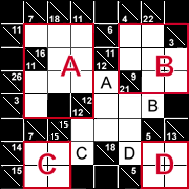6. Permutations With permutations we verify every possible combination for a run based on the candidates. The aim is to eliminate candidates until only one is left. In this example all the candidates are written in each box. One of the permutations for the down run 11 in 2 with boxes B and E is the following: If B = 2 then E = 9 (11-2). 9 doesn’t occur in the candidates for E so B cannot be equal to 2. The permutations for B and E are: B=4 + E=7 and B=5 + E=6. The remaining candidates for B are 45 and for E are 67. For the across run 11 in 3 the candidates for C are 12 and for E are 67. The possible combinations with these numbers are 137, 146 and 236. In other words, the candidates for D are 34.If we apply this technique to the boxes A and H then we get the following situation (see picture). If we search for the combinations in our table for the across run 16 in 3 with the boxes FGH then we see that only one solution is possible F8+G2+H6. We also immediately notice that there is only one solution for the down run 10 in 3, namely A=1 + D=3 + H=6. The remaining boxes can be filled in with the ‘solitary box’ technique. B=5, C=1, E=7 and I=1. Contact Us to request a free 'Member Login' so you can download our complete catalogues.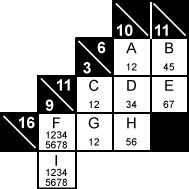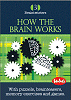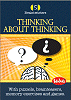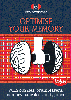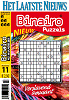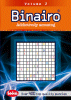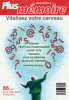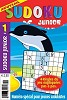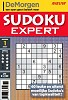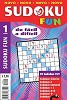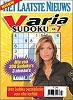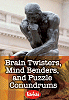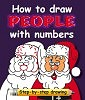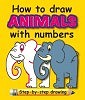Home   |  About Us   |  Contact Us PeterFrank, Postbus 11, 9830 Sint-Martens-Latem, Belgium | Copyright © 2022 - All rights reserved.